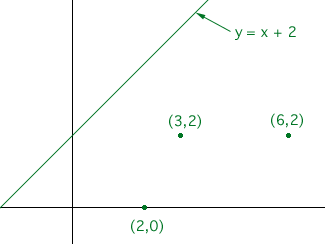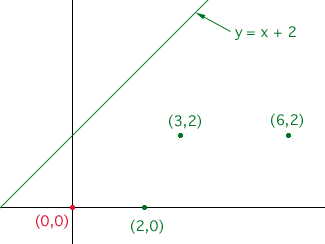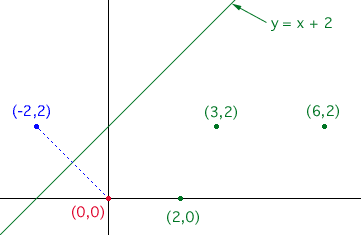ok: find the verticles of a triangle after it is translated 2 units to the left and then is reflected across the graph of y=x+2. The original verticles of the triangle are (2,0), (3,2), and (6,2). Then i have to explaub my answer and what i did. I am very bad at math and i need this bonus to boost up my grade but i am lost. please please please can you help me?? Hi, I would first draw a reasonably accurate diagram.I want to move one point, the point (2,0). You can move the other two points. First translate (2,0), 2 units to the left, to the red point in the diagram.Now draw a line segment, through (0,0), perpendicular to y = x + 2. Extend this line segment an equal distance on the other side of y = x + 2 to the point (-2,2). This is where (2,0) lands after the translation and reflection.Now move the other two points in a similar fashion. Penny Go to Math Central